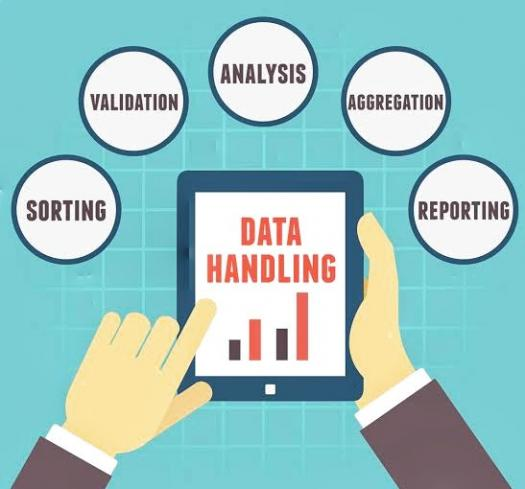# Quiz On Data Handling

30 Questions | Total Attempts: 38Settings• 1.
Pictorial representation of data using symbols is known as_____
• A.

Bar Graph

• B.

Pictograph

• C.

Histogram

• D.

Histograph

• 2.
Double bar graphs display ______ sets of data simultaneously.
• A.

0

• B.

1

• C.

2

• D.

3

• 3.
_________ gives the number of times a particular entry occurs.
• A.

Organisation of Data

• B.

Collection of Data

• C.

Representation of Data

• D.

Frequency Distribution Table

• 4.
In grouped data, each of the group is called:
• A.

Class Interval

• B.

Data Size

• C.

Frequency

• D.

Limit

• 5.
If 20-30 is the class interval of grouped data, then lower class limit is_____
• A.

10

• B.

20

• C.

30

• D.

25

• 6.
The difference between the upper class limit and lower class limit of a class interval is called_____
• A.

Frequency

• B.

Width

• C.

Grouping

• D.

Regrouping

• 7.
The width or size of the class interval 30-40 is_____
• A.

-10

• B.

10

• C.

30

• D.

40

• 8.
If a coin is flipped in the air, what is the probability of getting a tail?
• A.

0

• B.

1

• C.

0.25

• D.

0.50

• 9.
A bag has 4 red balls and 4 green balls, what is the probability of getting a red ball randomly?
• A.

0

• B.

1

• C.

0.25

• D.

0.5

• 10.
If a die is thrown in air, the probability of getting even numbers is______
• A.

0

• B.

1

• C.

0.5

• D.

0.25

• 11.
A probability near 1 is _______.
• A.

Even

• B.

Unlikely

• C.

Likely

• D.

Certain

• 12.
A bag has 3 red marbles, 2 blue and 4 yellow. What is the probability of pulling a red?
• A.

Certain

• B.

Even

• C.

Unlikely

• D.

Likely

• 13.
Change this decimal to a percent. 0.528
• A.

528%

• B.

52.8%

• C.

5.28%

• D.

0.528%

• 14.
What is the probability of spinning the spinner and having it land on RED?
• A.

33%

• B.

50%

• C.

75%

• D.

100%

• 15.
What is the probability of rolling a cube and having land as an odd number?
• A.

0

• B.

1/4

• C.

1/2

• D.

3/4

• 16.
What is the probability of rolling a cube and having it land as a six?
• A.

1/6

• B.

5/6

• C.

1/3

• D.

3/6

• 17.
The probability of it raining today is 30%. What is the probability that it will NOT rain?
• A.

30%

• B.

70%

• C.

100%

• D.

0%

• 18.
Probability can be written as_____
• A.

Decimal

• B.

Fraction

• C.

Percentage

• D.

All Of The Above

• 19.
The probability of drawing a 10 from a standard deck of 52 cards is _____
• A.

1/52

• B.

2/52

• C.

4/52

• D.

13/52

• 20.
A set of all possible outcomes is known as_____
• A.

List

• B.

Space

• C.

Sample Space

• D.

Event

• 21.
Probability of any event is always more than 1.
• A.

True

• B.

False

• 22.
Histograms and Histographs are Same.
• A.

True

• B.

False

• 23.
Probability of a Certain Event is 1.
• A.

True

• B.

False

• 24.
Another Name for Circle Graph is Pie Chart.
• A.

True

• B.

False

• 25.
When Two Dice Are Rolled Together, sample space has 6 events.
• A.

True

• B.

FalseBack to top